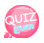# QUIZGUM

## Coding Class

This time, let's look at the alert window.

Have you ever seen a small box on the web while surfing the web?

You do not have it?The box shown in the image above is the alert window. It is used as one of ways to tell something

Today, let 's learn how to pop up this alert window.

### JavaScript

`    alert('hi');`

If you put the above source into the script tag, the box will appear and the text hi will be printed.

When entering a string, enclose it in quotes.

Let's try source and see how it looks.

### JavaScript

```<script>
</script>```

Just put the JavaScript source in the script tag as above.

Now, let's type the full source below.

```<!DOCTYPE html>
<html>
<meta charset="utf-8" />
<title>JavaScript</title>
<script>
</script>
<body>
</body>
</html>```

As above, an altar window opens and shows hi. ^ - ^

It is also possible to perform arithmetic operations in the alert() window. For example, writing 5 + 3 will show the result, 8.

### JavaScript

Example: alert (5 + 3); Computes 5 + 3 because it is recognized as a number.
False: alert ('5 + 3'); '' Because it is recognized as a character string and is not operated.

I write this operations as I wrote below. Do not enclose the quotation marks in a string, as the computer must recognize it as a number.

So let's write the actual source.

```<!DOCTYPE html>
<html>
<meta charset="utf-8" />
<title>JavaScript</title>
<script>
</script>
<body>
</body>
</html>```

If you look at the above results, you can see that the result 8 is displayed in the alert window.

So what should I do to show the phrase 12 * 6 = 72 in the Alert window?

Then I'll write this.

12 * 6 = is enclosed in quotes because it is represented by a string.

72 follows the string "12*6=", and it represents the operation "12*6"

Then '12 * 6 = 'as above, we need to connect this to 12 * 6. For the connection, we need +. Therefore,

`alert('12*6='+12*6);`

So let's write the actual source.

```<!DOCTYPE html>
<html>